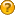###Author Topic: Rounded Cube  (Read 1903 times)

####miron123

• byte
•• Posts: 16##### Rounded Cube
« on: September 17, 2012, 10:38:32 am »
Hello.
I have a small problem. In the last 4 month i tried to create a cube with rounded edges. First I tried OpenGl ES and finally I found my way to jpct-ae. Now its a imported obj file.
Problem: I try to start new Activities based on the cube side the user is looking at, but I don t want to check for all 4 rotation matrices of each cube side. So I wanted to ask if someone knows a better solution. I heard Quaternion are a good way to go, but I don t realy have a clue how they work.
Code: [Select]
`public class MyMath { public static int getVisibleCubeSide(SimpleVector vector){ int vectorX = (int) Math.round(vector.x); int vectorY = (int) Math.round(vector.y); int vectorZ = (int) Math.round(vector.z);     int[][] posibilities = new int; //klassenbuch posibilities = 0; posibilities = 0; posibilities = 1; posibilities = 2; //studenplan posibilities = 0; posibilities = 1; posibilities = 0; posibilities = 1; //countdown posibilities = 0; posibilities = 0; posibilities = -1; posibilities = 0; //Lehrer posibilities = 0; posibilities = -1; posibilities = 0; posibilities = 5; //Trainer posibilities = 1; posibilities = 0; posibilities = 0; posibilities = 4; //new termine posibilities = -1; posibilities = 0; posibilities = 0; posibilities = 3; //checks if the parameter vector equals one of the vectors above for(int i = 0; i < 6; i++){ if(vectorX == posibilities[i] && vectorY == posibilities[i] && vectorZ == posibilities[i]){ Log.i("side", "v" + vector.toString()); Log.i("side", "s: " + posibilities[i]); return posibilities[i]; } } Log.i("side", "" + -1); return -1; }`Thats how i calculate my cube side atm. but it s not always correct cause i only check for one rotation matrix for each side but each side has 4 rotation matrices.
So I hope somebody has a good idea how to calculate the cube side based on matrices.

####EgonOlsen

••